# How to Decide the Perfect Distance Metric For Your Machine Learning ModelDistance metric is a key factor in many machine learning (ML) algorithms. ML itself is commonly used to recognize distinct images and derive information from them. Technologies like face recognition and recommendation engines use it to deliver information to whatever users seek. However, in order to help algorithms understand the similarities between content, users need to maintain a certain distance from the subject. This gap or distance is known as distance metric.

The perfect distance metric helps in learning the input data pattern by calculating the similarity between data points and concluding informative decisions. It adds to the performance of the classification, clustering, and information retrieval process that are involved in supervised as well as unsupervised machine learning algorithms.

This article will discuss the various distance metrics, examine the mathematics behind them, and look at their use in ML algorithms to help you choose the perfect one for your ML model.

## Euclidean distance

Euclidean distance is a widely used distance metric. It works on the principle of the Pythagoras theorem and signifies the shortest distance between two points.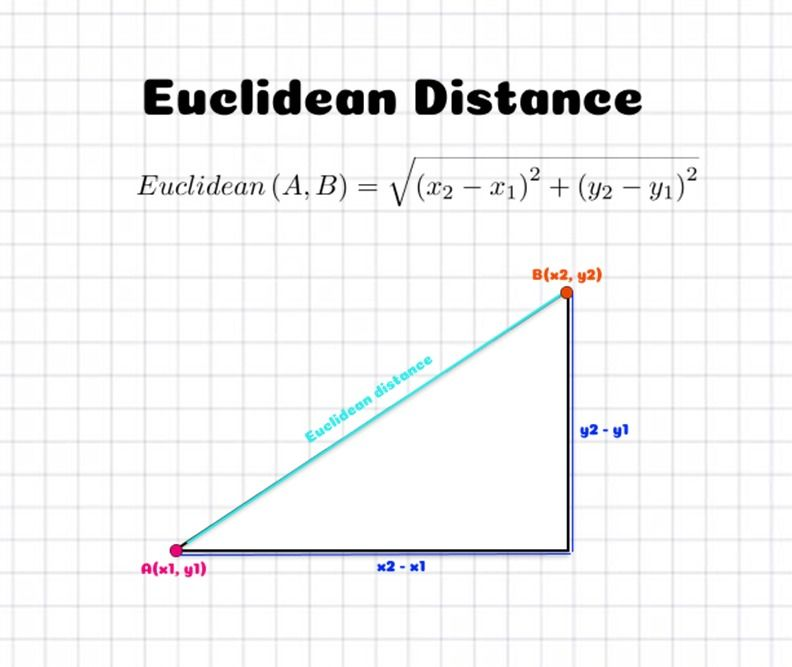Image source: https://cdn-images-1.medium.com/max/800/1*ZrwEraj9S-u_KOWdKWc8sQ.png

Mathematically, for an n-dimensional space and (pi, qi) as data points, the perfect distance metric is calculated by: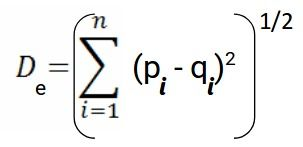Image source: https://cdn-images-1.medium.com/max/1067/1*lHQbNP1Grabz0ViiPM9uow.png

Euclidean distance is used in many machine learning algorithms as a default distance metric to measure the similarity between two recorded observations. However, the observations to be compared must include features that are continuous and have numeric variables like weight, height, salary, etc. Users mostly opt for it to calculate the distance between two rows of data that consists of numerical values.

Now, if you had to code for the same in Python, it would be:

`import numpy as np`
`#Function to calculate the Euclidean Distance between two points`
`def euclidean(p,q)->float:`
`distance = 0`
`for index, feature in enumerate(p):`
`d =(feature - q[index])**2`
`distance = distance + d`

`return np.sqrt(distance)`

Google Maps is an excellent example of the Euclidean distance metric, which calculates the distance between two real-valued vectors.

## Manhattan distance

The Manhattan distance or the cityblock distance is used to calculate the distance between two coordinates in a grid-like path.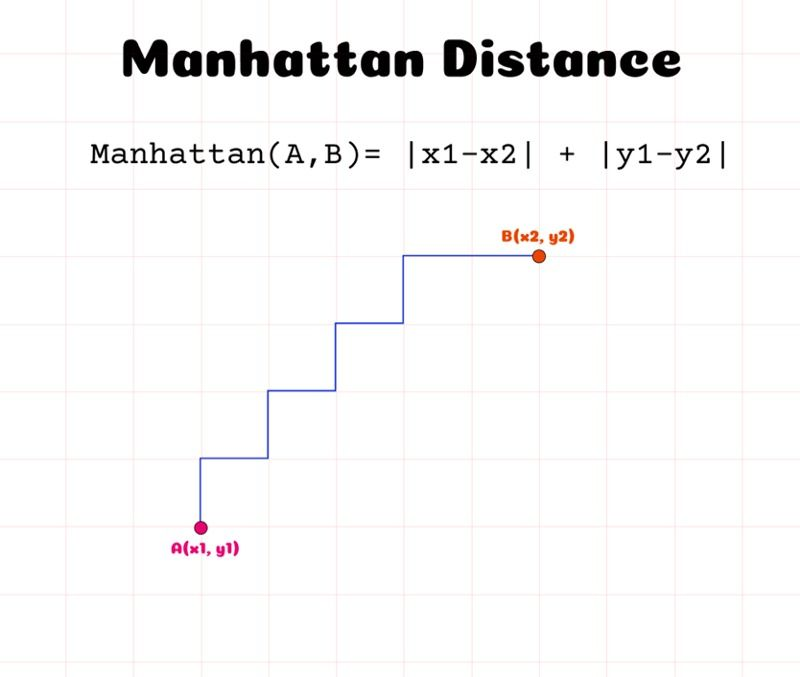Image source: https://cdn-images-1.medium.com/max/800/1*-xXnL0liqSl-flWgCTFbiw.png

It is a more effective metric in cases when discrete or binary attributes are present in the dataset as the path derived from the given values of attributes can be perceived in reality.

The generalized formula to calculate the Manhattan distance in an n-dimensional space is: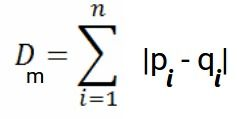Image source: https://cdn-images-1.medium.com/max/1067/1*9A3Ni7-UQ4NjDQd4e1kXaA.png

Here’s how you can write the code to find this metric:

`import numpy as np`
`#Function to calculate the Manhattan Distance between two points`
`def manhattan(a,b)->int:`
`distance = 0`
`for index, feature in enumerate(a):`
`d = np.abs(feature - b[index])`
`distance = distance + d`

`return distance`

Note that in the case of floating attributes present in the dataset, the Manhattan distance fails to represent the optimal distance. You can use it for high dimensional data but it is not preferred over Euclidean distance, performance-wise.

Manhattan distance is also known as taxicab distance. The thought behind this name is directed at the distance that a taxi driver would choose to travel from one point to another in the shortest way possible.

## Minkowski distance

This distance metric is a generalization of the Euclidean and Manhattan distance metrics. It determines the similarity of distances between two or more vectors in space. In machine learning, the distance metric calculated from the Minkowski equation is applied to determine the similarity of size.

It is also renowned as the p-norm vector, which represents the order of the norm. It allows the addition of the parameter p that enables the measurement of different distances that are to be calculated.

Here’s the formula to calculate the perfect distance metric: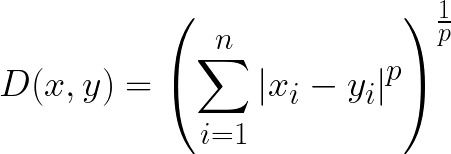Image source: https://miro.medium.com/max/451/0*HLw6nHhJescO2YJN

where

p=1 for Manhattan distance
p=2 for Euclidean distance
p=infinity for Chebyshev distance

Note: Chebyshev distance is the maximum distance along each axis in a given space.

Here’s the function for calculating Minkowski distance in Python:

`def minkowski_distance(a, b, p):`
`return sum(abs(e1-e2)**p for e1, e2 in zip(a,b))**(1/p)`

#OR

`from scipy.spatial import minkowski_distance`
`dist = minkowski_distance(row1, row2)`
`print(dist)`

## Hamming distance

This distance metric is the simplest of all. Hamming distance is used to determine the similarity between strings of the same length. In a simple form, it depicts the number of different values in the given two data points.

For example:

A = [1, 2, 5, 8, 9, 0]
B = [1, 3, 5, 7, 9, 0]

Thus, Hamming distance is 2 for the above example since two values are different between the given binary strings.

Here’s the generalized formula to calculate Hamming distance:

d = min {d(x,y):x, y∈C, x≠y}

Hamming distance finds application in detecting errors when data is sent from one computer to another. However, it’s important to ensure data points of equal length are compared so as to find the difference. Moreover, it is not advised to use Hamming distance to decide the perfect distance metric when the magnitude of the feature plays an important role.

Here’s the function to calculate Hamming distance in Python:

`def hamming_distance(a, b):`
`return sum(abs(e1 - e2) for e1, e2 in zip(a, b)) / len(a)`

#OR

`from scipy.spatial.distance import hamming`
`dist = hamming(row1, row2)`
`print(dist)`

These are the distance metrics you will use the most when dealing with machine learning models. Now that you know the features of each, which to use for different datasets, and how to code them in Python, you can decide on the perfect distance metric for your machine learning model.### Press

What's up with Turing? Get the latest news about us here.### Blog